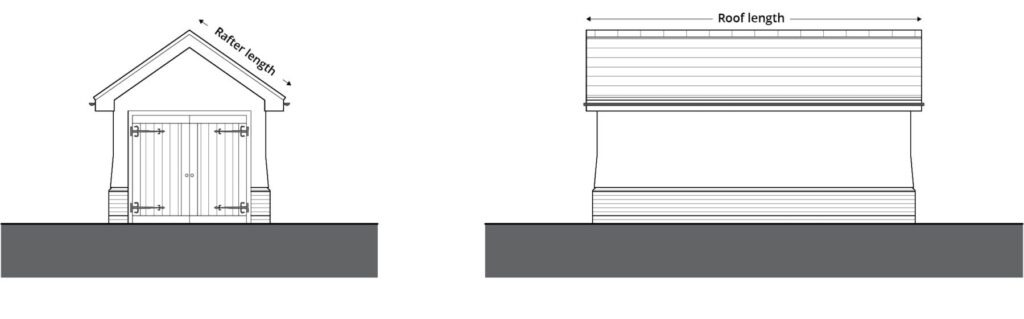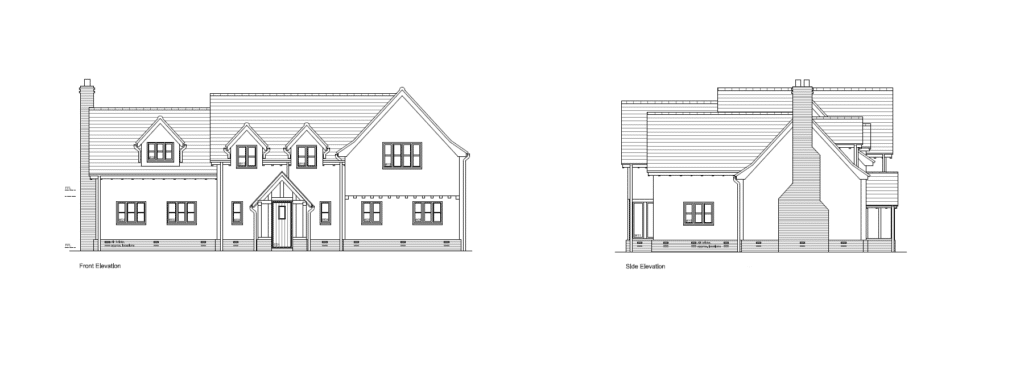# How do I calculate my roof area?

How do I calculate my roof area?
March 22, 2019In Tips

## Calculating the area of your roof

If you’re embarking on a new project, be it a new build or renovation, you may need to know how to calculate a roof area so you can estimate how many roof tiles you will need. So where do you start?

### Get organised

Before you start, you will need to have access to any plans for your project to make sure you have the correct dimensions of the proposed new roof area. Your architect should be able to provide you with a copy of the plans.

If your plans do not include a roof plan, you should still be able to calculate the quantities from elevation drawings.

### Which measurements do I need?

There are some key measurements that you will have to know in order to calculate your roof area. These are:

The length of your roof: This is the length of the eaves.
The rafter length: This is calculated my measuring from the eaves to the ridge.### Let’s get calculating

Once you have these measurements, you can calculate your roof area as follows:

Roof width (m) x rafter length (m) = half roof area (m²)

The above calculation estimates the area of half of your roof so you will need to double this to get a final estimate of the full roof area. This also assumes that there are no chimneys or other areas which are not tiled, or to be tiled. This does not allow for ridge tiles and other fittings that will be needed.

TOP TIP: Always add an additional 2.5% of tiles on to the total to allow for breakages and cuts.

The above is a simple calculation, which allows for a basic roof with no chimneys, pitched roofs which meet, areas of the roof which are not tiled or a combination of differing tile types. Where you need to calculate a complicated roof area, such as that pictured below, it is also best to ask for help.